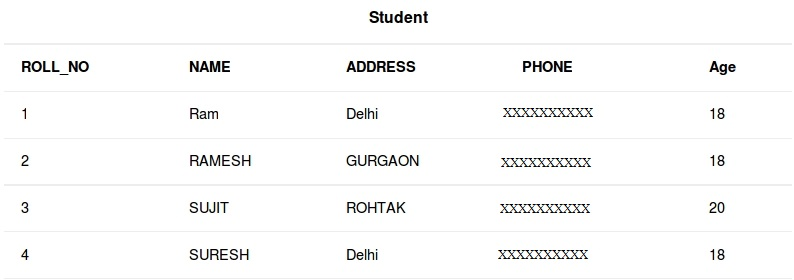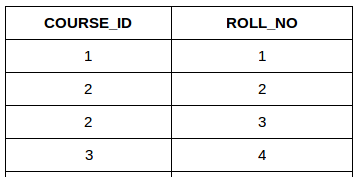# SQL | Join (Cartesian Join & Self Join)

SQL| JOIN(Inner, Left, Right and Full Joins)

• CARTESIAN JOIN
• SELF JOIN

Consider the two tables below:StudentCourse1. CARTESIAN JOIN: The CARTESIAN JOIN is also known as CROSS JOIN. In a CARTESIAN JOIN there is a join for each row of one table to every row of another table. This usually happens when the matching column or WHERE condition is not specified.
• In the absence of a WHERE condition the CARTESIAN JOIN will behave like a CARTESIAN PRODUCT . i.e., the number of rows in the result-set is the product of the number of rows of the two tables.
• In the presence of WHERE condition this JOIN will function like a INNER JOIN.
• Generally speaking, Cross join is similar to an inner join where the join-condition will always evaluate to True

Syntax:

```SELECT table1.column1 , table1.column2, table2.column1...
FROM table1
CROSS JOIN table2;

table1: First table.
table2: Second table
```

Example Queries(CARTESIAN JOIN):

• In the below query we will select NAME and Age from Student table and COURSE_ID from StudentCourse table. In the output you can see that each row of the table Student is joined with every row of the table StudentCourse. The total rows in the result-set = 4 * 4 = 16.
```SELECT Student.NAME, Student.AGE, StudentCourse.COURSE_ID
FROM Student
CROSS JOIN StudentCourse;
```
1. SELF JOIN: As the name signifies, in SELF JOIN a table is joined to itself. That is, each row of the table is joined with itself and all other rows depending on some conditions. In other words we can say that it is a join between two copies of the same table.Syntax:
```SELECT a.coulmn1 , b.column2
FROM table_name a, table_name b
WHERE some_condition;

table_name: Name of the table.
some_condition: Condition for selecting the rows.
```

Example Queries(SELF JOIN):

```SELECT a.ROLL_NO , b.NAME
FROM Student a, Student b
WHERE a.ROLL_NO < b.ROLL_NO;
```

If you like GeeksforGeeks and would like to contribute, you can also write an article using write.geeksforgeeks.org or mail your article to review-team@geeksforgeeks.org. See your article appearing on the GeeksforGeeks main page and help other Geeks.